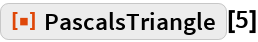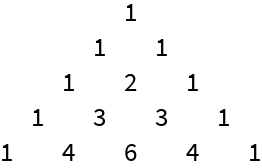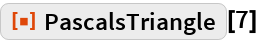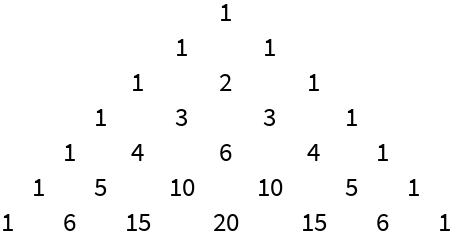Function Repository Resource:

PascalsTriangle

Display a given number of rows in Pascal's triangle

Contributed by: Paco Jain (Wolfram Research)
 ResourceFunction["PascalsTriangle"][n] creates a grid representing the first n rows of Pascal's triangle.

Details and Options

ResourceFunction["PascalsTriangle"][n] returns an n-by-2n+1 grid whose elements are either integers or the empty string "".

Examples

Basic Examples

Display the first five rows of Pascal's triangle:

 In:=Out=Display the first seven rows of Pascal's triangle:

 In:=Out=Requirements

Wolfram Language 11.3 (March 2018) or above